Bridging the Gap- Modeling and Methods in Maths(Part 2)

there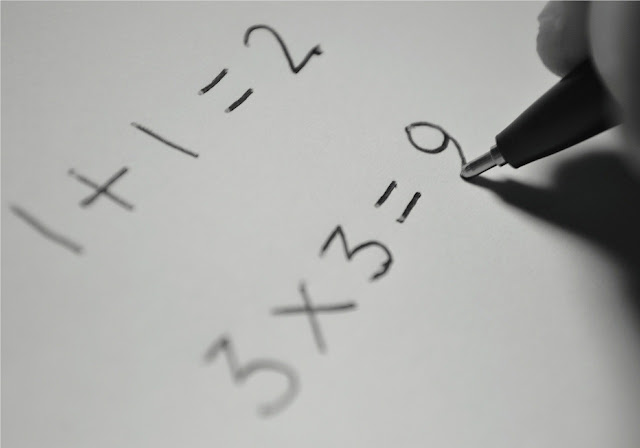Doing maths

Understanding Maths requires one to be able to analyse the question and choose the most appropriate method to solve the question. To be able to decide on which method  to use the child has to be able to  identify the crux of the  problem before he/she is able to slowly show the required workings to solve the problem.

Using the Model Method

From Primary 1 students are taught to draw models to solve Long Answer Questions. This is the  method that is “promoted” as it is supposedly the easiest method to explain how you can derive the answer. ( And NO you are not allowed to even use Algebra to explain yourself)

Question 1

Sammy and Gerald had an equal number of stamps at first. Sammy then gave away 20 of this stickers to his friend Winston, and Sammy Gerald bought another 12 stamps. In the end, Gerald had thrice as many stickers as Sammy. Find the number of stamps Sammy had at first.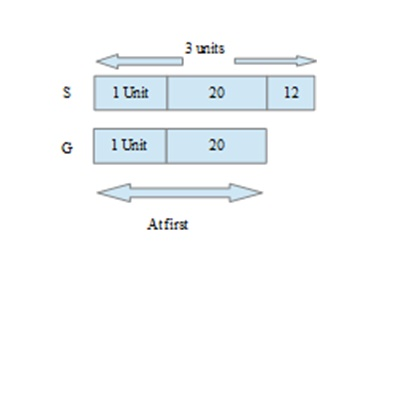Therefore 2 units 20 + 12

1 unit 32 2

1 unit → 16

Number of stamps Gerald had at first = 1 unit + 20

16 + 20

= 36

Gerald had 36 stamps at first.

If you notice the type of model drawn differs from the type of models drawn in lower primary.  It is more complex and you are comparing more then two different items involving multiple steps to solve the question.

Looking at a Pattern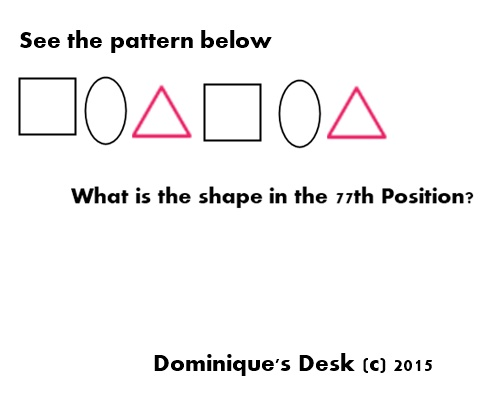Identifying the Pattern

How do you solve this problem?

Firstly you would need to identify the pattern.  Based on the diagram above you would notice that there are 3 different shapes – Square, Oval and Triangle.  The pattern repeats itself after the 3rd shape.

Step 1- Find out the number of “groups of 3” which are possible from 1- 77

77  3 = 25.66 ~ 25 times ( 25 R 2)

25x 3 = 75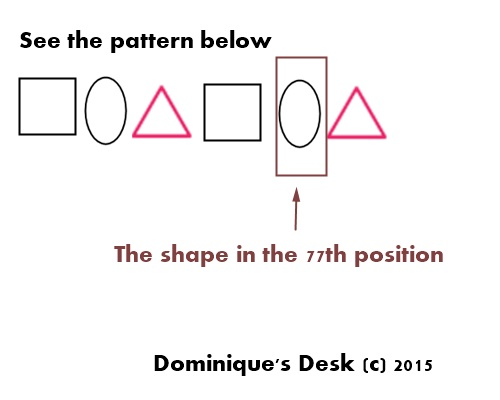It does take a lot of practice to be able to solve a mathematical question easily.   It’s fortunate that Maths is one of Monkey boy’s stronger subject and it doesn’t take him too much effort to understand the concepts which needs to be applied to the various questions.

How to you reinforce the learning of Maths for your kids?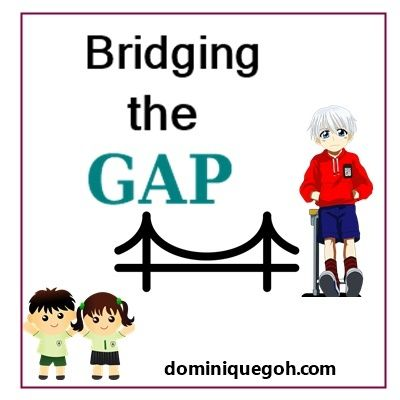Post in this series

9) Bridging the Gap- Upper and Lower Primary Science

10) Bridging the Gap-  Moving from Lower to Upper Primary

11) Bridging the Gap- Moving from Lower to Upper Primary ( Part 2)